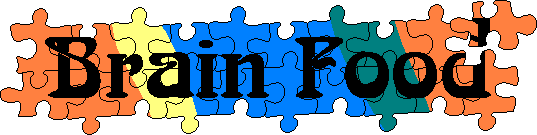Main      Site Guide### Word Ends

In each of these puzzles, a word starts and ends with the same letter. Can you figure out what the word is?

_lini_

Solution

_aure_

Solution

_hipsa_

Solution

_ngor_

Solution

_aci_

Solution

_rues_

Solution

_ysti_

Solution

_loh_

Solution

_ayhe_

Solution

_ustfu_

Solution

_rys_

Solution

_oya_

Solution

_yni_

Solution

_ylo_

Solution

_allo_

Solution

_urre_

Solution

_riti_

Solution

_idter_

Solution

_rui_

Solution

_enti_

Solution

_ren_

Solution

_aoba_

Solution

_wix_

Solution

_luf_

Solution

_wili_

Solution